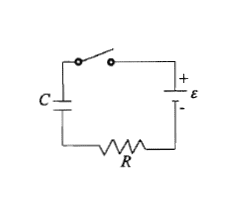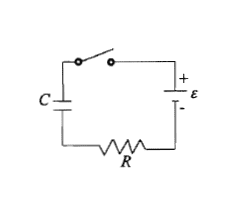# Capacitance, t=0 and t=infinity

## Homework Statement

1. Referring to the picture, switch S1 is closed at t=0.
a) What is the current in the circuit loop at t=0 and t=∞?
b) What is the voltage on C at t=0 and t=∞? (4M)## Homework Equations

When charging,
1.) ε - iR - q/c = 0
2.) max. current = -ε/R
3.) max. charge = C*ε
4.) q = Qfinal(1 - e^(-t/(RC))
5.) i = (Qfinal/RC)*e^(-t/(RC))

## The Attempt at a Solution

a) In fact I don't understand what t = 0 means. So when t = 0, is there any current yet?
For t = ∞, I tried to sub t = ∞ to equation 5) and use equation 3) to find the Qfinal. But it doesn't seem correct. Should I do it this way?
b) When t = 0, voltage on C = ε - iR? I assume the current has already thrown through the circuit when t = 0?
For t = ∞, should I sub t = ∞ into equation 5)? But it seems i = ∞ then? doesn't seem correct.(Plus, how do we calculate the resistance of a capacitor? When I google it, I found someone said it's assumed to be zero. So in calculation, I assumed its resistance to be zero?)

Last edited:

ehild
Homework Helper

## Homework Statement

1. Referring to the picture, switch S1 is closed at t=0.
a) What is the current in the circuit loop at t=0 and t=∞?
b) What is the voltage on C at t=0 and t=∞? (4M)## Homework Equations

When charging,
1.) ε - iR - q/c = 0
2.) max. current = -ε/R
3.) max. charge = C*ε
4.) q = Qfinal(1 - e^(-t/(RC))
5.) i = (Qfinal/RC)*e^(-t/(RC))

## The Attempt at a Solution

a) In fact I don't understand what t = 0 means. So when t = 0, is there any current yet?
Initially, the switch is open. No curent flows and there is no charge on the capacitor. You start measuring time at the instant when you close the switch. Current starts to flow, but there is no charge on the capacitor yet.
For t = ∞, I tried to sub t = ∞ to equation 5) and use equation 3) to find the Qfinal. But it doesn't seem correct. Should I do it this way?

What did you get for the current at t = ∞?

b) When t = 0, voltage on C = ε - iR? I assume the current has already thrown through the circuit when t = 0?
For t = ∞, should I sub t = ∞ into equation 5)? But it seems i = ∞ then? doesn't seem correct.You should know how the voltage on the capacitor is related to the charge on it, and that the charge is increased in the rate of current flow..
Current flows onto the capacitor till the voltage across the capacitor is less than the electromotive force ε. At t=0, the charge is 0. What is the current then according to equation 1.)?
As the charge accumulates on the capacitor, the capacitor voltage Uc increases and the voltage across the resistor Ur decreases. As i=Ur/R , the current decreases. After very long time the capacitor voltage become very close to the emf and the currents tends to zero.

Try to sketch how q and i changes with time according to 4.) and 5.).